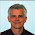### Globs Contest meets Parabolas (Lesson 3.4)

This is the glob array I posted for the contest on 10/31/08 and here is my video that describes one solution using linear equations. In order to get the highest score possible you need to to maximize the number of globs you hit with each equation.

I was hoping to get 5 globs with one and I came close with my first shot, but it missed (1,-1). The same was true for shot #2. Here’s all 5 of my shots. (I made the figure below with Geometer’s Sketchpad.)So I managed to get 37 points. I’m not sure that I can do better using linear equations. But what if could use parabolas? Mr. Jacobs introduces us to parabolas in lesson 4. He defines it as the graph of y equals x squared. In set 1 he explores the patterns that help us to move the parabola along the y axis and also how to draw it “upside down” by making the coefficient of the x-squared term negative. In the next video clip I describe more about graphing parabolas.

Here’s the three parabolas I used to get my score of 61 points. I used the vertex form of the equation to draw each parabola. To draw the parabola so its orientation is "perpendicular" to the original parabola switch the x and y in the equation.

To help you use the vertex form of the equation to draw parabolas more effectively try out the Interactive parabola applet at http://www.mathwarehouse.com/quadratic/parabola/interactive-parabola.php

PS. If you go to the website above and want to see a perfectly awful example of a math video game click on their banner promoting the game or click here.ClimeGuy said…
Latest PC & Mac versions of Green Globs is available at http://greenglobs.netClimeGuy said…
See my Green Globs Contest blog entry here.

More details about the contest are at this site.